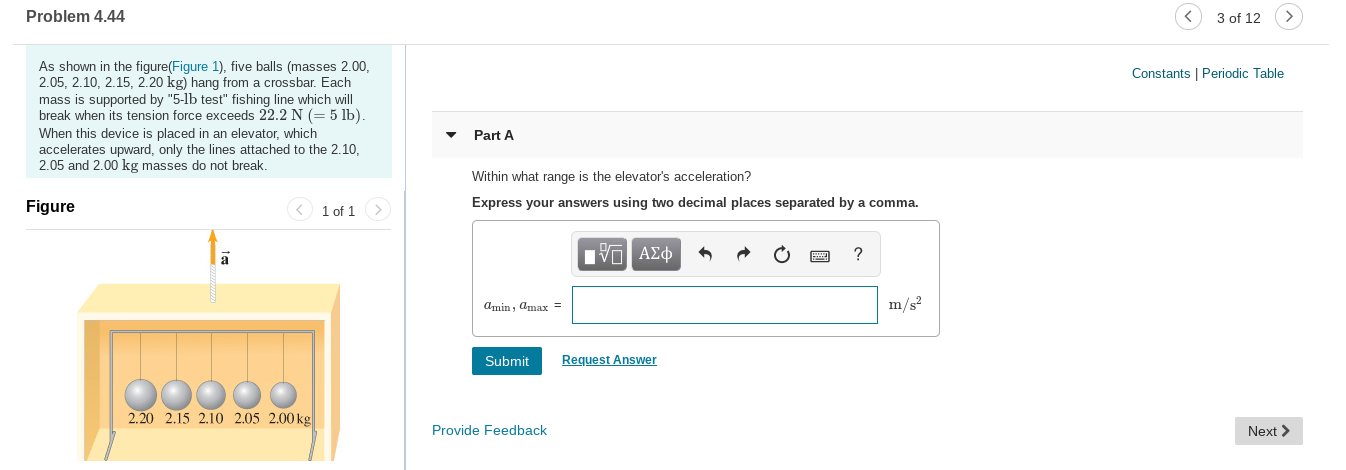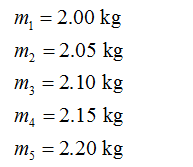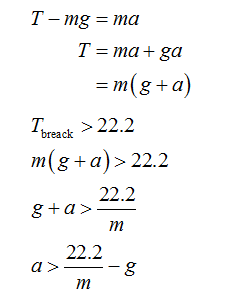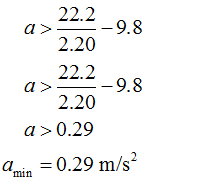# Problem 4.443 of 12As shown in the figure(Figure 1), five balls (masses 2.00,2.05, 2.10, 2.15, 2.20 kg) hang from a crossbar. Eachmass is supported by "5-lb test" fishing line which willbreak when its tension force exceeds 22.2 N (= 5 lb).When this device is placed in an elevator, whichaccelerates upward, only the lines attached to the 2.10.Constants | Periodic TablePart A2.05 and 2.00kg massesdo not break.Within what range is the elevator's acceleration?Express your answers using two decimal placesseparated bya comma.Figure<1 of 1V ΑΣφAmin, Amax =m/s2SubmitRequest Answer2.20 2.15 2.10 2.05 2.00 kgProvide FeedbackNext >

Question
87 viewshelp_outlineImage TranscriptioncloseProblem 4.44 3 of 12 As shown in the figure(Figure 1), five balls (masses 2.00, 2.05, 2.10, 2.15, 2.20 kg) hang from a crossbar. Each mass is supported by "5-lb test" fishing line which will break when its tension force exceeds 22.2 N (= 5 lb). When this device is placed in an elevator, which accelerates upward, only the lines attached to the 2.10. Constants | Periodic Table Part A 2.05 and 2.00 kg masses do not break. Within what range is the elevator's acceleration? Express your answers using two decimal places separated by a comma. Figure < 1 of 1 V ΑΣφ Amin, Amax = m/s2 Submit Request Answer 2.20 2.15 2.10 2.05 2.00 kg Provide Feedback Next > fullscreen
check_circle

Step 1

Given data:

Minimum tension, Tmin = 22.2 N

Masses:Step 2

From the Newton’s second law, the net force on a mass in a lift which is moving upward can be written:Step 3

For the minimum acceleration, it is necessary to break off  heaviest mass, therefore:...

### Want to see the full answer?

See Solution

#### Want to see this answer and more?

Solutions are written by subject experts who are available 24/7. Questions are typically answered within 1 hour.*

See Solution
*Response times may vary by subject and question.
Tagged in

### Physics